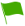# C4.2 Reaction rates and Reversible Reactions

1.What is the effect of light on the rate of reaction?

2.Define optimum conditions

3.Mention the factors which affect Equilibrium

4.What do you expect when you add up a catalyst such as Manganese (IV) oxide in a chemical reaction?

5.What is the effect of temperature on the rate of reaction?

6.Mention the factors affecting the rate of reaction

7.A substance that alters the rate of reaction of a chemical reaction but remains chemically unchanged at the end of the reaction is called?

8.What is the effect of adding a catalyst such as Manganese (IV) oxide to the rate of reaction?

9.What is the effect of surface area on the rate of reaction?

10.What is the effect of pressure on the rate of reaction of a gas chemical reaction?

11.Explain the reason why a change in pressure has no effect on those reactions in which both reactants and products are either solids or liquids

12.Mention some of the properties which are observed when reactants are consumed to form products per unit time

13.How does the amount of time taken affect the rate of reaction?

14.A change in pressure has no effect on those reactions in which both reactants and products are either solids or liquids even if they are in an equilibrium state. True or False

15.Define Collision Theory

16.The reaction rate is a measure of how much of the reactions are consumed or how much of the products are formed per unit time. True or False

17.A change in pressure will only affect equilibrium in reactions involving gases. True or False

18.How does pressure affect a system of gas which is in equilibrium?

19.A catalyst has no effect on the position of equilibrium it only alters the rate at which the equilibrium is attained. True or False

20.State Le Chatelier’s Principle

21.How does the change in concentration affect equilibrium?

22.Discuss photochemical reactions

23.Define reversible reactions

24.The minimum energy required by the reacting particles to cause a successful collision to form products is referred to as

25.What is the effect of change of temperature on equilibrium

26.When the concentration of the particles of the reactants is high how does it affect the rate of reaction

27.What is the effect of pressure on the rate of reaction of a solid and liquid chemical reaction?